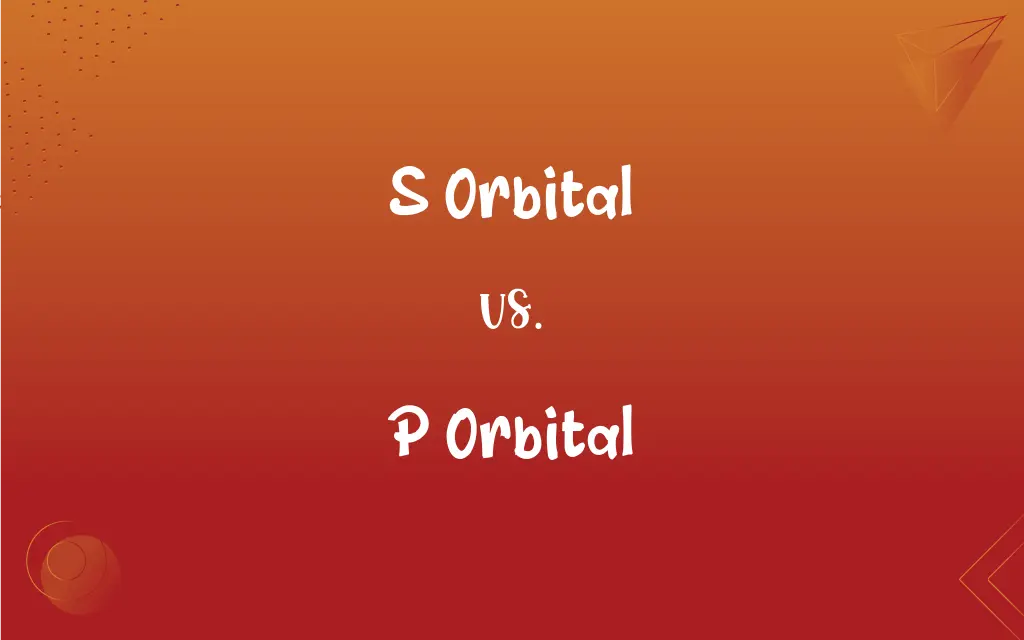# S Orbital vs. P Orbital

The main difference between S Orbital and P Orbital is that S Orbital is the spherical shaped orbital in the atom, whereas P Orbital is the dumbbell-shaped orbital in the atom.## Key Differences

S orbital is defined as the spherical shaped orbital in an atom, whereas p orbital is dumbbell-shaped orbital in an atom.
S orbitals contain a maximum of 2 electrons in its shell; conversely, p orbitals contain a maximum of 6 electrons in its orbitals.
In s orbitals, the maximum number of sub orbitals is 1, whereas, in p orbital, the maximum orbital number is 3.
S orbital is the low energy orbitals; on the other hand, p orbitals are high energy orbitals.
In s orbitals, the electrons revolve in spherical shaped; on the flip side, in p orbitals, electrons revolve in dumbbell-shaped orbitals.
In s orbitals, the number of lobes is zero, whereas, in p orbitals, there are few numbers of lobes.

## Comparison Chart

### .

It is defined as the spherical shaped orbital in an atom.
It is defined as the dumbbell-shape orbital in an atom.

### Energy Level

S orbital has a low energy level orbital and can easily donate the electron.
P orbital has a high energy level and cannot easily donate an electron.

### Angular Nodes

S orbital has zero angular nodes.
P orbital has some number of nodes.

### Maximum Electrons

S orbital can hold only 2 electrons.
P orbital can hold only 6 electrons.

### Number of Orbitals

S orbital has no sub orbitals.
P orbitals have three sub orbitals.

### Angular Momentum

The angular momentum of s orbital is zero.
The angular momentum of p orbitals is 1.

### Number of Lobes

In s orbitals, there is zero number of lobes.
In p orbitals, there are few numbers of lobes.

### S Orbital vs. P Orbital

In the s orbital, the energy level is low, whereas, in p orbital, the energy level is high. S orbital has zero angular nodes; on the other hand, p orbital has a different angular node. In s orbital, the maximum two electrons which it can hold in its orbital, whereas in p orbital, it can hold six are in its orbital. S orbital is defined as the spherical shaped orbital in the atom, whereas p orbital is defined as the dumbbell-shaped orbital in the atom.

In s orbital, there are no subshells or sub orbitals, whereas, in p orbital, there are three sub orbitals. In s orbital, there is zero angular momentum; on the other hand, in p orbital, the angular momentum is 1. In s orbital, there is no number of lobes, whereas, in p orbitals, there are few numbers of lobes. The filling of the electron is firstly filled in s orbital, whereas the filling of the electron is second in p orbitals.

It is difficult to remove the electron from s orbital due to high ionization energy, whereas it is easy to lose the electron from p orbital due to low ionization energy. S orbital is present quit near to the nucleus of the atom, whereas p orbital is present quite away from the nucleus. In s orbital, only two electrons revolve around the nucleus, whereas in p orbital, six electrons revolve above the s orbital and around the nucleus of an atom.

### What is S Orbital?

It is defined as the spherical shape orbital in an atom. It is present near about above the nucleus in an atom. S orbital has low energy for hold of electrons. It can only hold 2 electrons. The two electrons revolve around the nucleus in spherical shapes. We required high energy to remove these two electrons. Under hybridization, this shell involves in head to head over to form different shapes of the molecule.

The electrons revolve continuously into orbital. The filling of the shell is started from s orbital. S orbital has zero angular momentum because of its spherical shape. S orbital has zero angular nodes due to its spherical shape. It is the first orbital in every atom. In s orbital, there is zero number of lobes. For example, in Hydrogen, the electronic configuration is 1s with 1 electron; it is an incomplete orbital.

But in Helium, the are 2 number of electrons, which is the complete orbital. The s orbital is present near the nucleus of an atom so that it is powerfully attracted by the nuclear charge. The atom is stable with this orbital.

### What is P Orbital?

It is defined as the dumbbell-shaped atomic orbital in an atom. It is present above the s orbital of an atom. It is more energized than s orbital. It holds six number electrons in its orbital in an atom. We do not require a large amount of energy to remove these electrons. In an atom, the p orbital electron is firstly involved in the bonding. By their bonding, the very little amount of energy is released.

Under hybridization, one of p sub orbitals forms head to head overlap, and the other two p sub orbitals form sideway overlap. P orbitals have few numbers of different angular nodes because it is present in 3 axes. The maximum number of electrons in p orbitals are 6 electrons. There are three sub orbitals in the p orbitals; every sub orbital contains 2 electrons in it.

Angular momentum of p orbitals is one because of its difference in its structure. It is oriented in the three axes, i.e., x, y, and z. For example, in the carbon atom, the maximum electron is six, the distribution is in the 1s 2s 2p. The p orbital is incompletely filled.